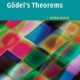# show that every propositional formula is equivalent to one using the connectives $\to$ and $\neg$

1,363

## Solution 1

As every propositional formula is equivalent to one using only $\land$ and $\neg$, it suffices to prove that $\land$ and $\neg$ can by written using $\to$ and $\neg$ resp. $\mid$.

We have \begin{align*} p \land q &\iff \neg(\neg p \lor \neg q)\\ &\iff \neg(p \to \neg q) \end{align*} and \begin{align*} \neg p &\iff \neg (p \land p)\\ &\iff p \mid p\\ p \land q &\iff \neg \neg (p \land q)\\ &\iff \neg (p \mid q)\\ &\iff (p \mid q)\Bigm| (p \mid q) \end{align*} So we are done.

## Solution 2

The heart of each problem is showing that all of the usual connectives can be obtained using the available ones. I’ll do a bit of each and leave the rest to you.

(a) We know (and you can easily check) that $p\to q$ has the same truth table as $\lnot p\lor q$. Thus, $\lnot p\to q$ must have the same truth table as $\lnot(\lnot p)\lor q$, which has the same truth table as $p\lor q$. That is, using only $\to$ and $\lnot$ I can simulate $p\lor q$ by $\lnot p\to q$. (Of course you should check this by writing out the truth tables!) To get $p\land q$, use de Morgan’s law to get it by combining $\lnot$ and $\lor$, now that you know how to get $\lor$.

(b) By definition $p\mid q$ has the same truth table as $\lnot(p\land q)$. Thus, $p\mid p$ must have the same truth table as $\lnot(p\land p)$, which in turn has the same truth table as $\lnot p$. (Check all this, of course, with the actual truth tables.) Thus, $p\mid p$ simulates $\lnot p$ using only the Sheffer stroke. So what does $(p\mid q)\mid(p\mid q)$ give you? If you don’t see it right away, write out the truth table. Once you have that and $\lnot$, getting the remaining connectives shouldn’t be too hard.

## Solution 3

A start: a) Use a truth table to show that $A\lor B$ is equivalent to $(\lnot A)\rightarrow B$.

b) Use a truth table to show that $\lnot A$ is equivalent to $A \mid A$, and observe that $A\lor B$ is equivalent $(A\mid A)\mid(B\mid B)$.

Share:
1,363Author by

### Khanak

Updated on June 12, 2022

•(a) Show that every propositional formula is equivalent to one using the connectives $\to$ and $\neg$.

(b) Show the same is true for the Sheffer stroke $|$ where $p\mid q$ means "either not $p$ or not $q$" or "not both $p$ and $q$".

•Pedro over 9 years
You can also use \neg to obtain $\neg$ and \to to obtain $\to$. Choose your favourite.
•Peter Smith over 9 years
If you are asking here, that strongly suggests you should be checking out some of the standard intro logic textbooks, which will explain this kind of thing in detail. Look up "expressive completeness" or "expressive adequacy" in the index. NB it is always a good move to get a couple of presentations from a couple of different books. Chapter 3 of Teller's Primer is freely available at tellerprimer.ucdavis.edu/pdf and is good. Or there's §11.7 of my Intro Formal Logic book. And dozens more of course.
•martini over 9 years
@JasonDeVito Thx.